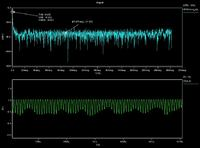# how to do transient noise analysis,pls

Status
Not open for further replies.

#### lhlbluesky

##### Banned
for a relative large circuit, how to do transient noise analysis in spectre?

if my circuit is large (for ex: 5000 transistors, and 6 sub-blocks), then how to run transient noise simulation in spectre? what is the procedure?

how to add the stimilus, and how to observe the results,pls?

can anyone give me some advice or related papers?

besides, for a cmos image sensor, how to simulate its total noise?

thanks all.

anyone would help me?

when you did transient simulation after you can convert the signal from time domain to frequency domain e.g. spectra . Thus you can view noise floor and compute it.

but for some case, transient noise is 200e- or 300e-, then what is the meaning of e-? and is it in time domain or frequency domain? and how to measure the transient noise in unit of e- for circuit simulation?
how to se up the transient noise parameter, such as noisescale, noisespeed and so on? what value would be best or better?
pls help me, any advice will be appreciated.

Measurement

Electronic noise is properly measured in relative to a reference, such as watts of power. Because noise is a random process, it can be characterized by stochastic properties such as its variance, distribution, and spectral density. The spectral distribution of noise can vary by frequency, hence its power density is measured in watts per hertz (W/Hz). Since the real power in a resistive element is proportional to the square of the voltage across the element, noise voltage (density) can be described by taking the square root of the noise power density, resulting in volts per root hertz (V/√Hz). Integrated circuit devices, such as op-amps commonly quote equivalent input noise level in these terms (at room temperature).

In atmospheric sound transmission, 'noise level is the noise power of the longitudinal sound wave relative to a point of reference.

In telecommunication engineering, noise level is usually measured in decibels (dB) for relative power or Watts for absolute power. A suffix is added to denote a particular reference base or specific qualities of the measurement. An example of acoustical noise-level measurement units is dB(A). Examples of electrical noise-level measurement units are dBu, dBm0, dBrn, dBrnC, and dBrn(f1 − f2), dBrn(144-line).

Noise levels are usually viewed in opposition to signal levels and so are often seen as part of a signal-to-noise ratio. Telecommunication systems strive to increase the electrical signal level to noise level ratio in order to effectively transmit data. In practice, if the transmitted signal falls below the level of the noise (often designated as the noise floor) in the system, data can no longer be decoded at the receiver. Noise levels in telecommunication systems are a product of both internal and external sources to the system including shot noise, thermal noise, and ambient electromagnetic interference.

Instead of noise speed or noise scale you can measure params such like THD ,SNR on specta
Heare is an exampleoh, thank you, i learned a lot.

For lhlbluesky,

So why is noise specified in unit of e- in sensor, and how does that get converted back and forth to V^2/Hz which is used in our noise simulation ?

in many cases the signal one measures is a charge, which is in turn converted to voltage with some conversion factor K (with units of inverse of a capacitance)
V=K Q
e.g. think of CCDs or CMOS sensors

you can refer your input rms noise (*) back to that original charge signal dividing it by K

(*) rms noise (measured in volts) is the square root of the integral of the noise power density spectrum (measured in V^2/Hz) over the noise bandwidth

Make sense.

The equation covers the V or e- unit. (although need to know C first)

I am still a little curious about the 1/Hz part of the unit of rms noise. Do you think this is dependent on the something else ?

tnoise is fairly new capability and you need to make sure your
PDK has the required model params, populated & proper. You
ought to be able to run a tnoise and massage the output data
(by FFT?) to compare to a believed-accurate noise curve just
to check capability / sanity.

Status
Not open for further replies.